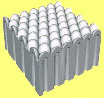## Research Papers in Physics and Astronomy## David Sellmyer Publications

#### Date of this Version

August 1978

Published by American Physical Society. Phys. Rev. B 18, 1377 (1978). http://prb.aps.org. Copyright © 1978 American Physical Society. Permission to use.

#### Abstract

We consider the random anisotropy model for amorphous magnetism by making a local-mean-field approximation (LMFA) on arrays of spin-one particles. Hysteresis loops and the temperature (T) dependence of several thermodynamic quantities are presented for various values of the ratio of the strength of the exchange (J) to the strength of the uniaxial anisotropy (D). Using the LMFA limits us to systems with a small number (N) of spins, of which we explicitly consider N=64, 216, and 1000. We assume periodic boundary conditions on a system with N1/3 spins along an edge, nearest-neighbor coupling of constant strength, and six nearest neighbors (as for a simple cubic lattice). For J>0 the free energy of spin-glass-like states is higher than that of corresponding states with remanent magnetization. The dependence of the coercive field (Bc) on J and D is discussed and the apparent discrepancy of Chi and Alben vis à vis Callen, Liu, and Cullen concerning the behavior of Bc for large D is clarified. A calculation of the temperature dependence of Bc is presented which is reminiscent of experimental results. This random anisotropy is found to give rise to a second peak in the specific heat for suitable values of D/J. The magnetic susceptibility (χT) is calculated for both positive and negative J and shows positive and negative paramagnetic Curie-Weiss temperatures, respectively. The slopes of the χT-1 (T) curves for T well above the critical temperature (Tc) have values that are roughly equal to 3/2, the value appropriate to D=0 and S=1. The local order parameter q is used to identify Tc, which correlates well with the critical temperature identified from other thermodynamic quantities. The presence of the random anisotropy is found to reduce Tc by up to about 25%. The results of several temperature-dependent calculations are summarized in a phase diagram and regions of paramagnetic, random ferromagnetic, and random antiferromagnetic (or spin-glass-like) behavior are identified.

COinS